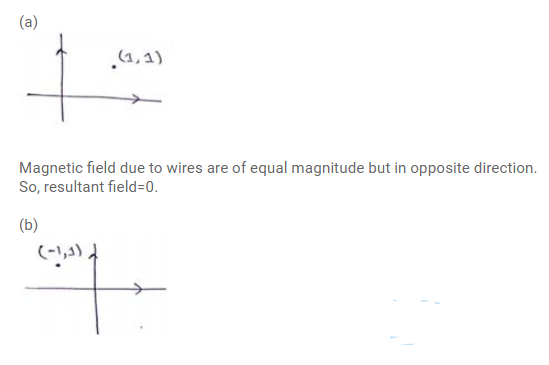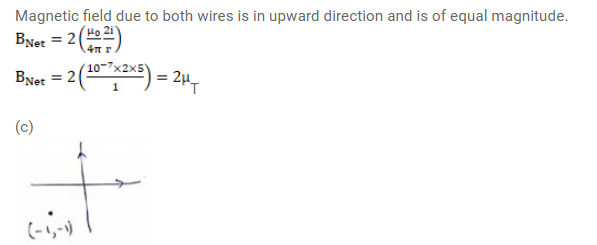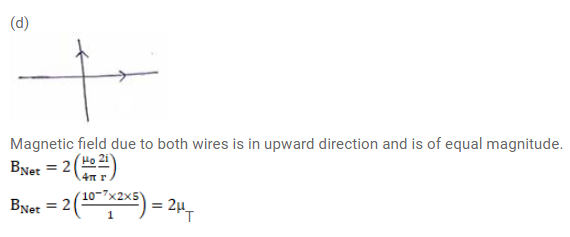# Two long, straight wires, each carrying a current of 5 A,

Question:

Two long, straight wires, each carrying a current of $5 A$, are placed along the $X$ - and Y-axes respectively. The currents point along the positive directions of the axes. Find the magnetic fields at the points

(a) (1m, $1 m)$,

(b) $(-1 m, 1 m)$,

(c) $(-1 m,-1 m)$,

(d) $(1 m,-1 m)$.

Solution:Magnetic field due to wires are of equal magnitude but in opposite direction. So, resultant field $=0$.